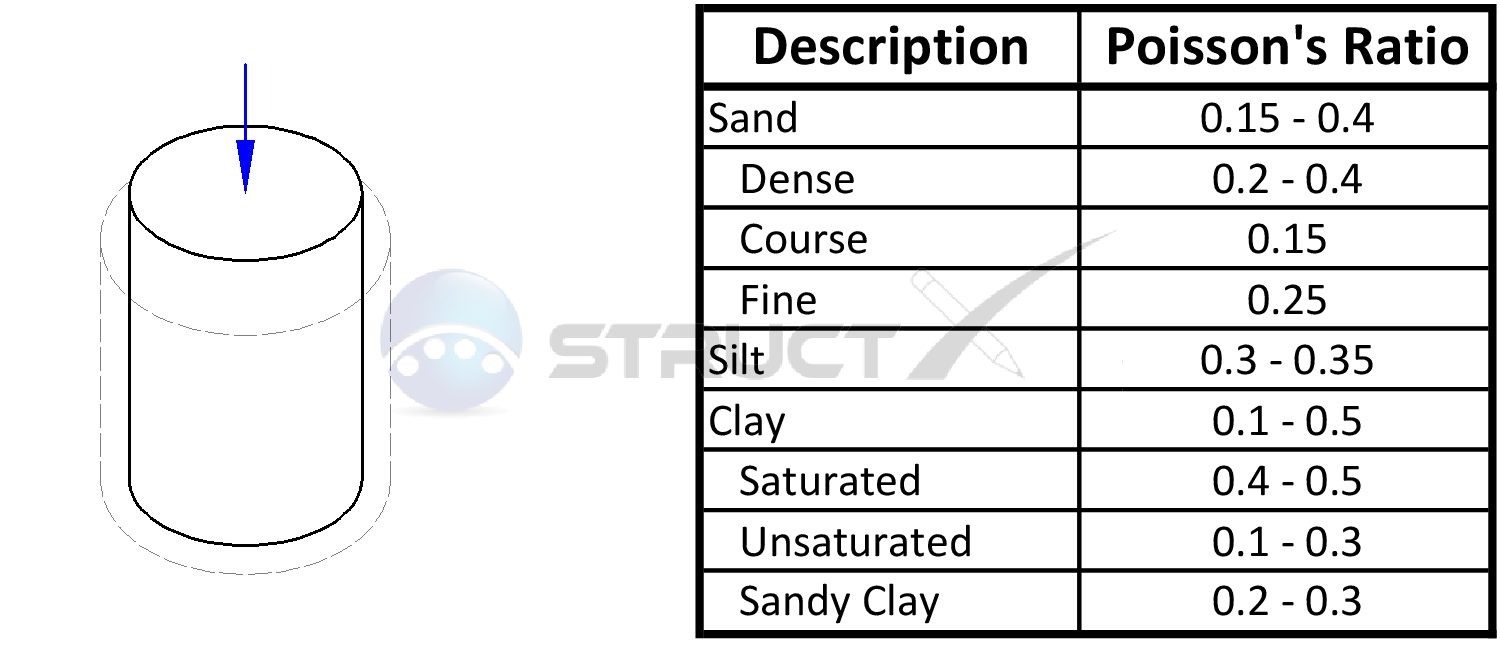# Typical Poisson's Ratio Values for Common Soil TypesMore Properties# Notation and Units

## Poisson's Ratio

Because Poisson's ratio can be considered to be unit-less, the above values can be used with both imperial and metric units of measure. As with all calculations care must be taken to keep consistent units throughout. A guide to Soil Types has been provided by StructX.

When a material is compressed or is subjected to a tensile force in one direction, it usually tends to either expand or contract in the other two directions perpendicular to the applied force. Mathematically, Poisson's ratio is the negative ratio of transverse to axial strain.

The Poisson's ratio of a stable, isotropic, linear elastic material cannot be less than −1.0 nor greater than 0.5 with the later being a value typically associated with a perfectly incompressible material. It should be noted that exceptions to the above values often occur and multiple values depending on the loading conditions are common.